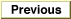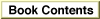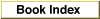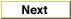# Legacy DocumentImportant: The information in this document is obsolete and should not be used for new development.

Inside Macintosh: QuickDraw GX Environment and Utilities /
Chapter 8 - QuickDraw GX Mathematics / QuickDraw GX Mathematics Reference
Mathematical Functions / Fixed-Point Operations

### FixedMultiply

You can use the `FixedMultiply` function to return the product of two numbers.

```Fixed FixedMultiply (Fixed multiplicand, Fixed multiplier);
```
`multiplicand`
The number to be multiplied by the multiplier.
`multiplier`

The number by which the multiplicand is to be multiplied.
function result
The product of two numbers.
##### DESCRIPTION
The `FixedMultiply` function multiplies two fixed numbers. The format of the `Fixed` number returned depends on the respective number formats of the multiplicand and multiplier. The operation has a bias of 16 bits; in general, the bias of the resulting number is the sum of the biases of the input numbers, shifted right by 16 bits. If either the multiplicand or the multiplier is `Fixed`, the result of the `FixedMultiply` function will be the same fixed-point format as the other parameter (`long`, `Fixed`, or `fract`).

Table 8-3 shows the bias of the product for different combinations of formats. The dashed line indicates that the resulting bias is not equivalent to long, fixed, or fract. Use the rules of the operation to determine it.
`FixedMultiply` product bias
longfixedfract
long---long---
fixedlongfixedfract
fract---fract---

##### SPECIAL CONSIDERATIONS
The `FixedMultiply` function does not pin its result in the case of an overflow; the result returned is modulo 65,536.© Apple Computer, Inc.
7 JUL 1996